07.14.13

Regularization – Predictive Modeling Beyond Ordinary Least Squares Fit

Posted in Linear Regression at 6:07 pm by Auro Tripathy

Introduction to Linear Functions and Regularization

A simple yet powerful prediction model assumes that the function is linear in the input even in cases where the input consists of hundreds of variables and the input variables far outstrip the number of observations. Such prediction models, known are linear regression/classification models, can often outperform fancier non-linear models.

The most popular method of estimation of the parameters (used interchangeably with the word, coefficients)  is the method of Ordinary Least Squares (OLS). The linear model can be written as

f(x) = βo + ∑Xβj

where j=1 to p, X is the input vector, and βjs are the unknown coefficients.

We solve for the coefficients β (βo1,…βp) that minimize the residual sum of squares (RSS).

RSS() = ∑(yi – βo - ∑Xijβj)2

where i=1 to N observations, j= 1 to p variables

Reasons why OLS estimation is often unsatisfactory are:

1. Large variance in prediction accuracy. A solution to improving the overall accuracy is to shrink (or set to zero) some of the coefficients. The overall effect is to prevent or reduce over-fitting.
2. With a large number of input predictors, one would like to determine a smaller subset that would exhibit the strongest effects so we see the big picture.

The process of regularization involves a family of penalty terms that can  be added to OLS to achieve the shrinkage (in the coefficients).

The Ridge penalty term shrinks the regression coefficients by introducing the complexity parameter, λ, the greater the value of λ, the greater the amount of shrinkage. By varying λ, the coefficients are shrunk towards zero (and to each other).

λ ∑ βj2 where j=1 to p

While the Ridge penalty does a proportional shrinkage, the LASSO penalty λ, translates each coefficients by a constant factor stopping at zero. LASSO also does feature-selection; if many features are correlated, LASSO will just pick one.

λ ∑ |βj|, where j=1 to p

Elastic Net penalty is a combination of the LASSO and Ridge regression penalty.

λ∑( α|βj| + (1 – α)βj), where j=1 to p

The first term encourages a sparse solution in the coefficients and the second term encourages highly correlated features to be averaged. The parameter α determines the mix of penalties and lies in the range of 0 and 1. With α set to 0, we get the Ridge penalty and with α set to 1, we get the LASSO penalty.

Example

We now demonstrate this with a example dataset with 204 binary attributes and 704 observations.

Getting the Data

The R snippet below will download the dataset  from where it is hosted. The data has been previously saved as an R object in the .rda format. We reload it back in to the R object, hiv.data.

load("hiv.rda", verbose=TRUE) #contains hiv.train & hiv.test

Visualizing the Data

The image function in R helps us visualize the dataset. You can see below the relatively strong correlation between the variables.  See the visualize.matrix function below.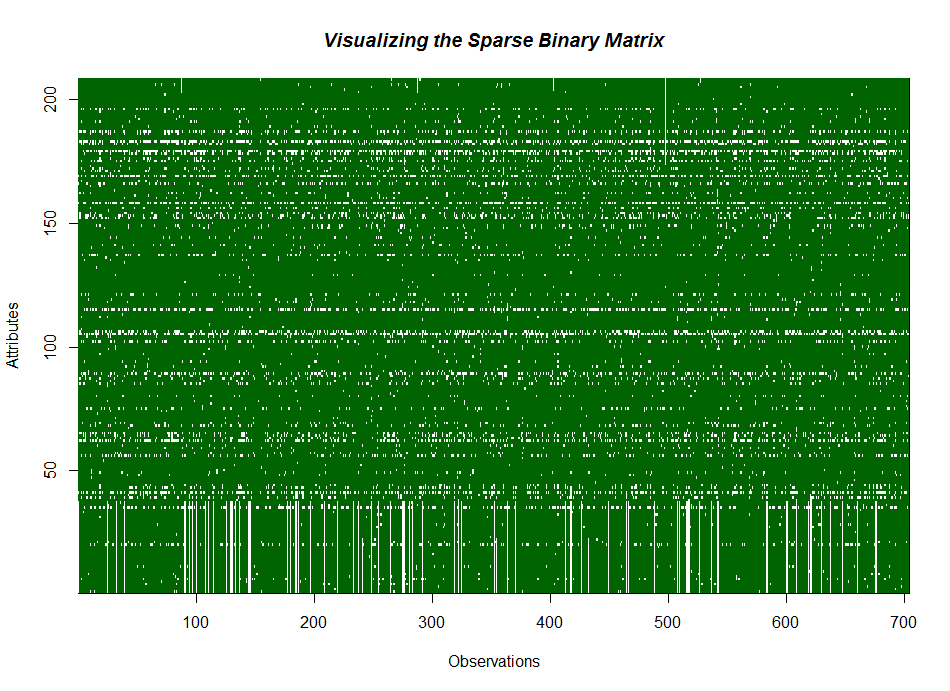Fitting/Plotting Data

The code snippet below shows the coefficient shrinkage is proportional to λ when we apply the Ridge penalty.

fit <- glmnet(hiv.train\$x,hiv.train\$y, alpha=0) #Ridge penalty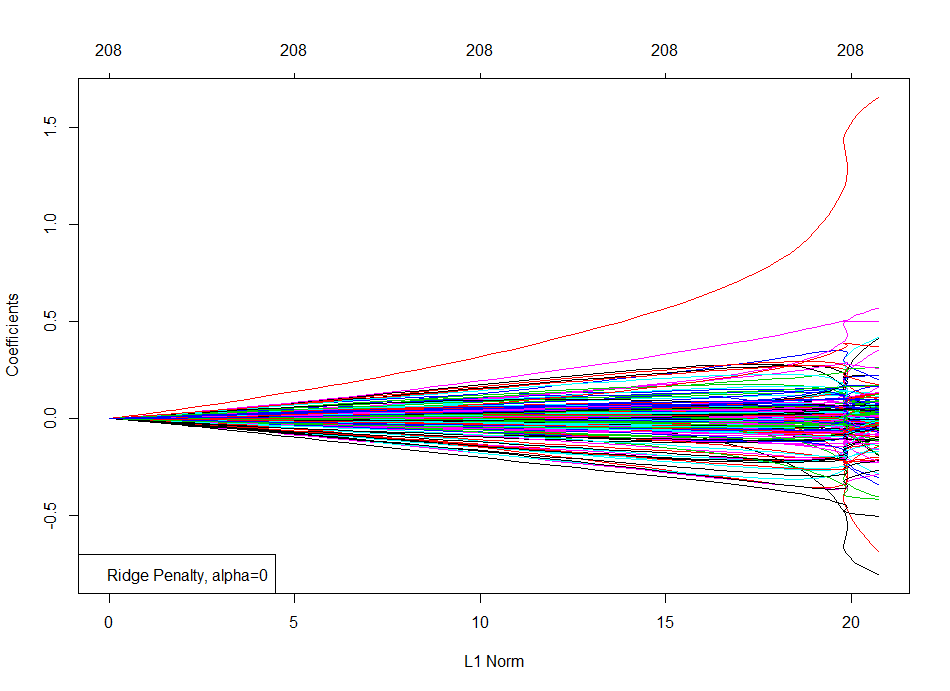The code snippet below shows that, with the LASSO penalty, the coefficient hit zero (unlike Ridge) as λ shrinks.

fit <- glmnet(hiv.train\$x,hiv.train\$y, alpha=1) #Lasso penalty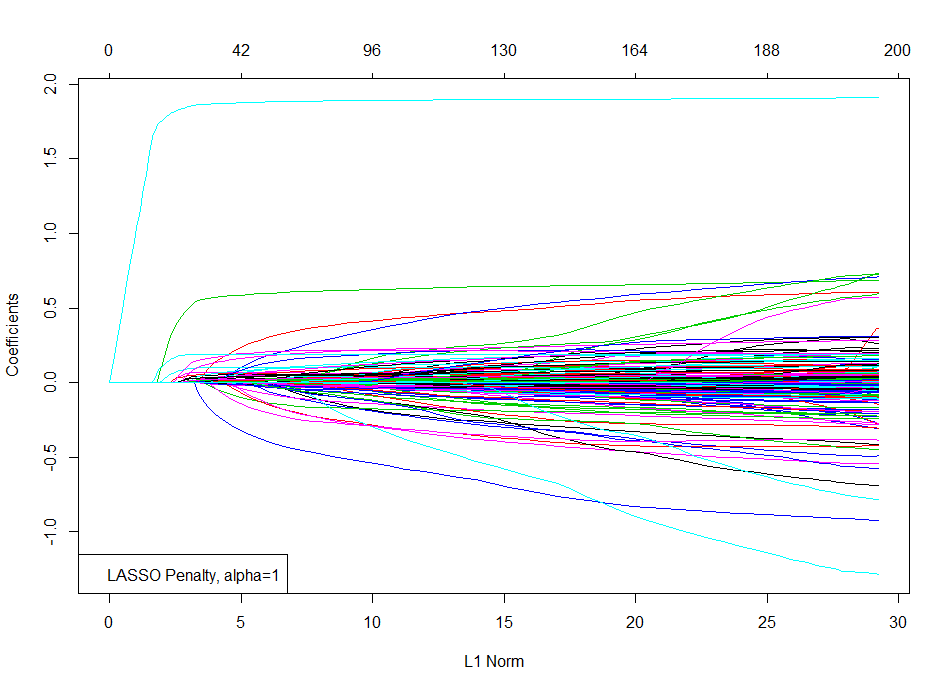The code snippet below shows a mix of the Ridge and LASSO penalties with the Elastic Net penalty for a specific value of  α=0.2 (could also be chose with cross-validation).

fit <- glmnet(hiv.train\$x,hiv.train\$y, alpha=0.2) #Elastic Net penalty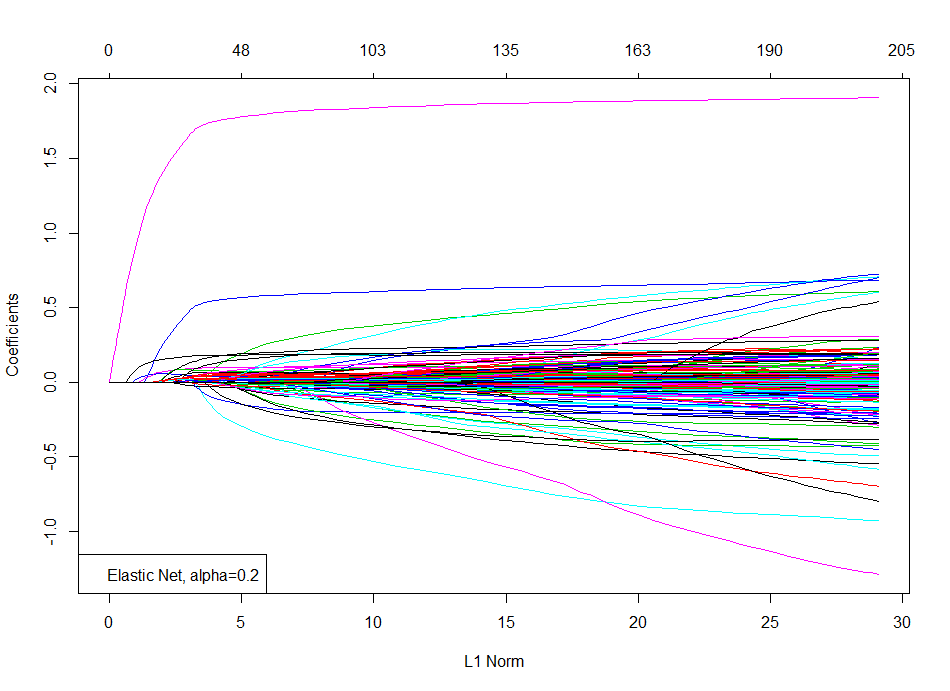Cross-Validation

Ten-fold cross-validation shows us that the number of active variables are approximately 30.

cv.fit <- cv.glmnet(hiv.train\$x,hiv.train\$y) #10-fold cross-validation plot(cv.fit) legend("topleft",legend=c("10-fold Cross Validation"))Predicting the Test Data with the Model

The code snippet below predicts the error at every value of  λ.

pred.y <- predict(fit, hiv.test\$x) #predict the test data
mean.test.error <- apply((pred.y - hiv.test\$y)^2,2,mean)
points(log(fit\$lambda), mean.test.error, col="blue",pch="*")
legend("topleft",legend=c("10-fold Cross Validation","Test HIV Data"),pch="*",col=c("red","blue"))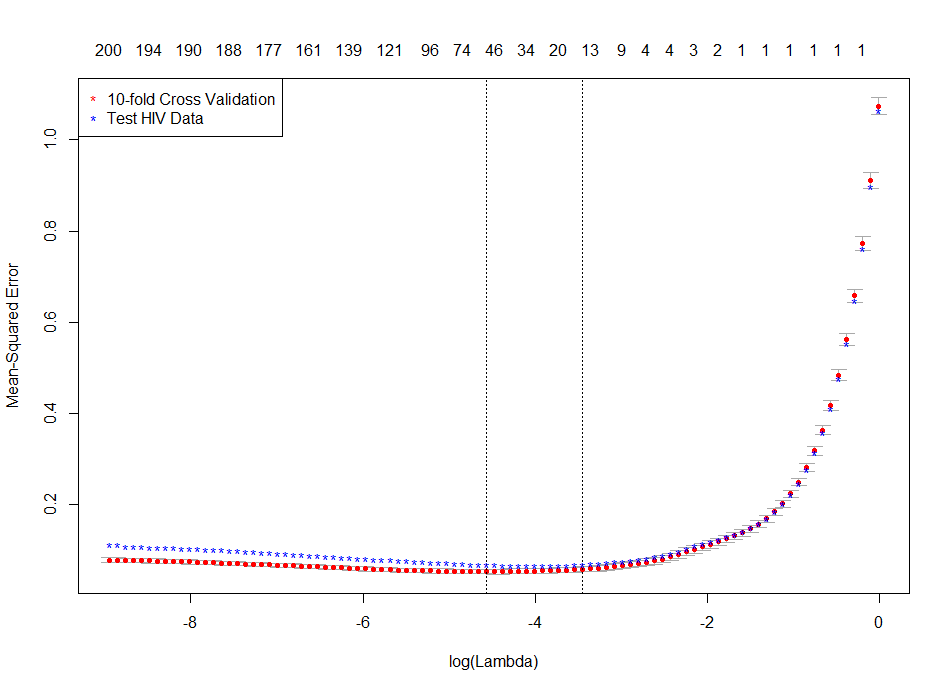Plotting the Regularization Path

The code snippet below shows the regularization path by plotting the coefficients against  (log of) λ. Each curve represents a coefficient in the model. As  λ gets smaller, more coefficients enter the model from a zero value. (see to the left).

plot(fit,xvar="lambda")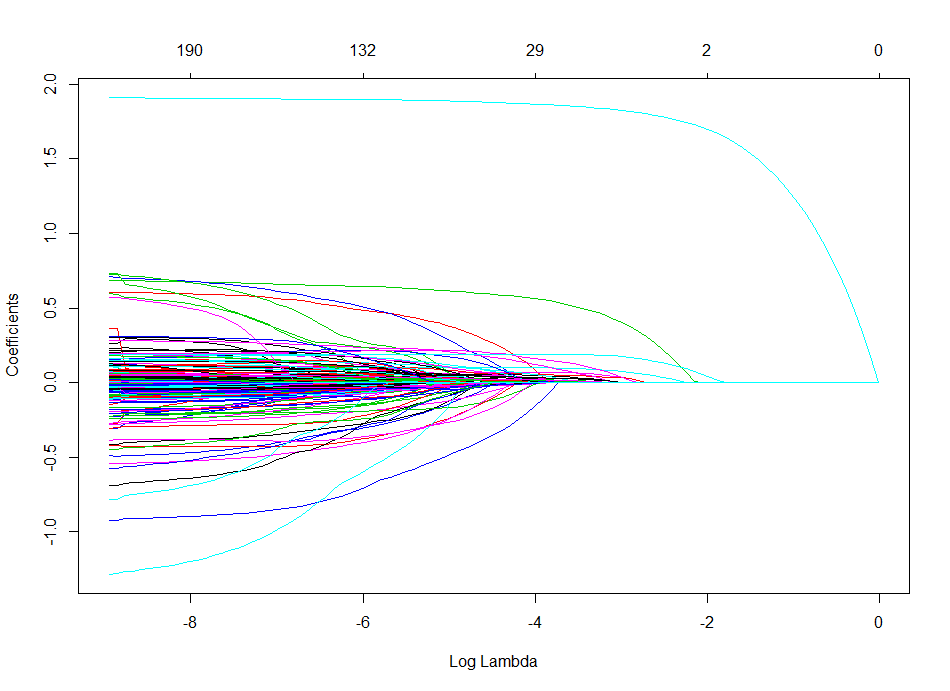Code

# Author Auro Tripathy, auro@shatterline.com
# Adapted from ...Trevor Hastie's talk
rm(list=ls())

visualize.matrix <- function(mat) {
print(names(mat))

image(1:nrow(mat\$x), 1:ncol(mat\$x), z=mat\$x,
col = c("darkgreen", "white"),
xlab = "Observations", ylab = "Attributes")

title(main = "Visualizing the Sparse Binary Matrix",
font.main = 4)
return (dim(mat\$x)) #returns the dimensions of the matrix
}

#---main---#
library(glmnet)
?glmnet
"hiv.rda", mode="wb")
verbose=TRUE) #contains hiv.train & hiv.test
visualize.matrix(hiv.train)
visualize.matrix(hiv.test)

print(length(hiv.train\$y)) #length of response variable

fit <- glmnet(hiv.train\$x,hiv.train\$y, alpha=0) #Ridge penalty
plot(fit)
legend("bottomleft",legend=c("Ridge Penalty, alpha=0"))

fit <- glmnet(hiv.train\$x,hiv.train\$y, alpha=1) #Lasso penalty
plot(fit)
legend("bottomleft",legend=c("LASSO Penalty, alpha=1"))

fit <- glmnet(hiv.train\$x,hiv.train\$y, alpha=0.2) #ElasticNet penalty
plot(fit)
legend("bottomleft",legend=c("Elastic Net, alpha=0.2"))

cv.fit <- cv.glmnet(hiv.train\$x,hiv.train\$y) #10-fold cross-validation
plot(cv.fit)
legend("topleft",legend=c("10-fold Cross Validation"))
pred.y <- predict(fit, hiv.test\$x) #predict the test data
mean.test.error <- apply((pred.y - hiv.test\$y)^2,2,mean)
points(log(fit\$lambda), mean.test.error, col="blue",pch="*")
legend("topleft",legend=c("10-fold Cross Validation","Test HIV Data"), pch="*", col=c("red","blue"))
plot(fit,xvar="lambda")
plot(fit,xvar="dev")

References

1. Prof Trever Hastie’s talk
2. The Elements of Statistical Learning: Data Mining, Inference, and Prediction,  Trevor Hastie , Robert Tibshirani , Jerome Friedman

06.23.13

Heart-Disease Predictor Using Logistic Regression

Posted in Linear Regression at 10:48 am by Auro Tripathy

Probability is the very guide of life.
- Cicero

Given a two-column dataset, column one being age and column two being the presence/absence of heart-disease, we build a model (in R) that predicts the probability of heart-disease at an age. For a realistic model we aught to have big datasets with additional predictor variables such as blood-pressure, cholesterol, diabetes, smoking etc. However, the one-and-only predictor variable we have is age and the sample-size is 100 subjects!

Plotting the data (see below) doesn’t really provide a clear picture of the nature of the relationship between heart-disease and age. The problem is that the response variable (presence/absence of heart disease) is binary.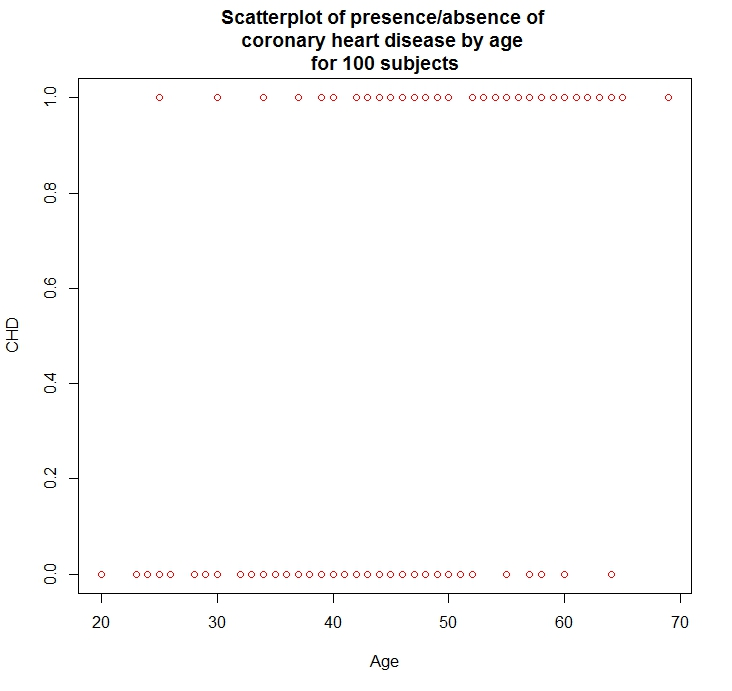Let’s create intervals of the independent variable (age) and compute the frequency of occurrence of the response variable (presence/absence of heart disease). You can get the table below  here.

A short and lucid tutorial in logistic regression is here (text) and here (video). The logistic curve is an S-shaped curve that takes the form,
y = [exp(b0 + b1x)] / [1 + exp(b0 + b1x)]

Clearly, the curve is non-linear, but the logit-transform makes it linear.
logit(y) = b0 + b1x

Thus, logistic regression is linear regression on the logit transform of y, where y is the probability of success at each value of x. Logistic regression fits b0 and b1, the regression coefficients.

The glm package in R is used to fit generalized regression models and can be used for logistic regression by specifying the family parameter to be binomial with the logit link like so:

> glm.out = glm(cbind(chd.present, chd.absent) ~ age.mean,
+               family=binomial(logit), data=frequency.coronary.data)

Plotting the fit shows us the close relationship between the fitted values and the observed values.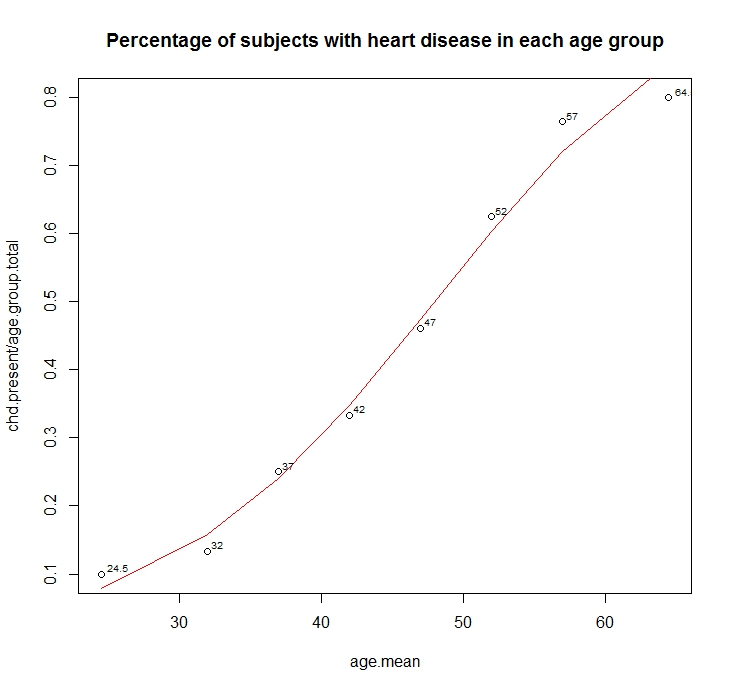Below is the R code that generated the plots.

rm(list=ls())
plot(CHD ~ Age, data=coronary.data, col="red")
title(main="Scatterplot of presence/absence of \ncoronary heart disease by age \nfor 100 subjects")

library(calibrate) #needed to label observation
frequency.coronary.data[,"age.mean"] <- (frequency.coronary.data\$age.start +
frequency.coronary.data\$age.end)/2
frequency.coronary.data <- frequency.coronary.data[, c(1,2,6,3,4,5)] #reorder cols
#With "family=" set to "binomial" with a "logit" link,
# glm( ) produces a logistic regression
glm.fit = glm(cbind(chd.present, chd.absent) ~ age.mean,
family=binomial(logit), data=frequency.coronary.data)

summary(glm.fit)
plot(chd.present/age.group.total ~ age.mean, data=frequency.coronary.data)
lines(frequency.coronary.data\$age.mean, glm.fit\$fitted, type="l", col="red")

textxy(frequency.coronary.data\$age.mean,
frequency.coronary.data\$chd.present/frequency.coronary.data\$age.group.total,
frequency.coronary.data\$age.mean, cx=0.6)
title(main="Percentage of subjects with heart disease in each age group")

Created by Pretty R at inside-R.org

References